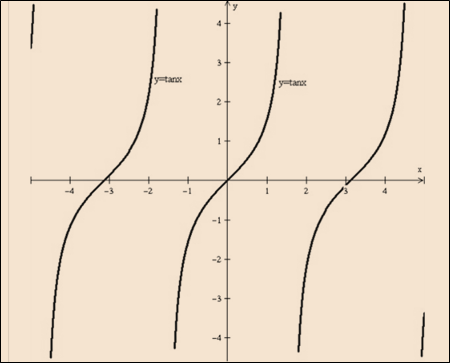# To graph: The following function with the help of the transformation: y = tan 2 x### Single Variable Calculus: Concepts...

4th Edition
James Stewart
Publisher: Cengage Learning
ISBN: 9781337687805### Single Variable Calculus: Concepts...

4th Edition
James Stewart
Publisher: Cengage Learning
ISBN: 9781337687805

#### Solutions

Chapter C, Problem 38E
To determine

## To graph: The following function with the help of the transformation:   y=tan2x

Expert Solution

### Explanation of Solution

Given information:

y=tan2x

Graph:Graph 1: y=tanxGraph 2: y=tan2x

Interpretation:

Need to draw a first graph of the function y=tanx as shown by the graph 1. Now, the given function y=tan2x is in form of the y=f(xc) . It means that need to shift the graph by y=f(cx) units on the right side or the graph of y=f(x) by a factor of c is shifted.

So compress horizontally the graph y=tanx the factor of 2 is used. It is shown by graph 2.

### Have a homework question?

Subscribe to bartleby learn! Ask subject matter experts 30 homework questions each month. Plus, you’ll have access to millions of step-by-step textbook answers!